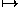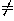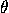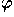# 11.1 Similarities

A transformation of space that preserves shapes is called a similarity. Every similarity of the plane is obtained by composing a proportional scaling transformation (also known as a homothety) with an isometry. A proportional scaling transformation centered at the origin has the form

(x,y,z)(ax,ay,az),

where a0 is the scaling factor (a real number). The corresponding matrix in homogeneous coordinates isIn cylindrical coordinates, the transformation is

(r,,z)(ar,,az).

In spherical coordinates, it is

(r,,)(ar,,).

Next: 11.2 Affine Transformations
Up: 11 Other Transformations of Space
Previous: 11 Other Transformations of SpaceThe Geometry Center Home Page

Silvio Levy
Wed Oct 4 16:41:25 PDT 1995

This document is excerpted from the 30th Edition of the CRC Standard Mathematical Tables and Formulas (CRC Press). Unauthorized duplication is forbidden.